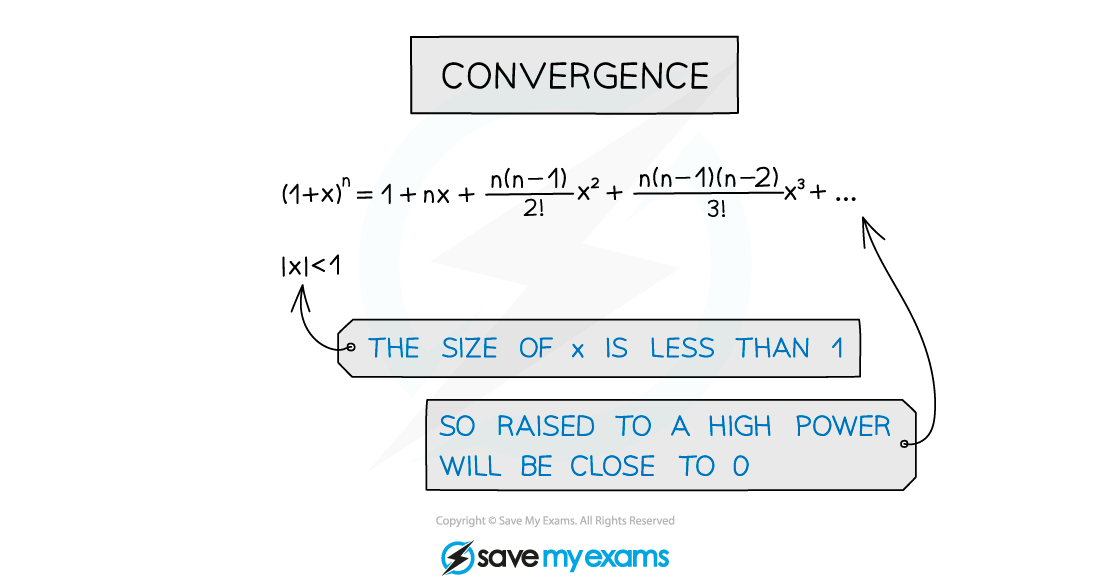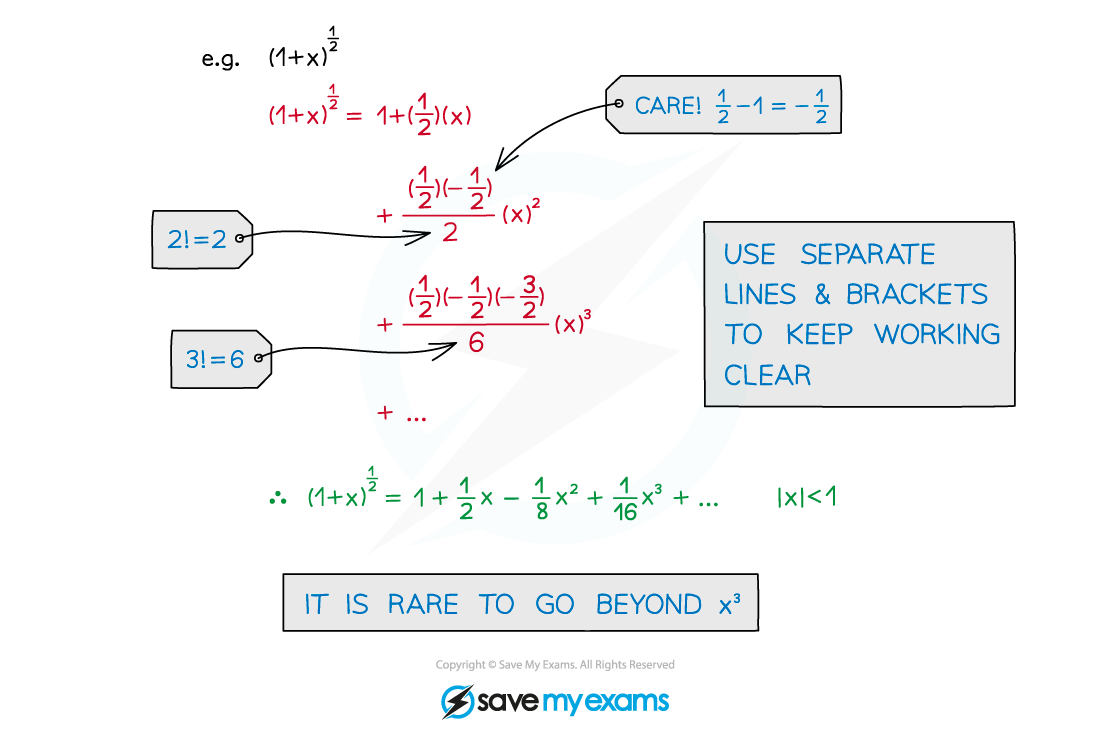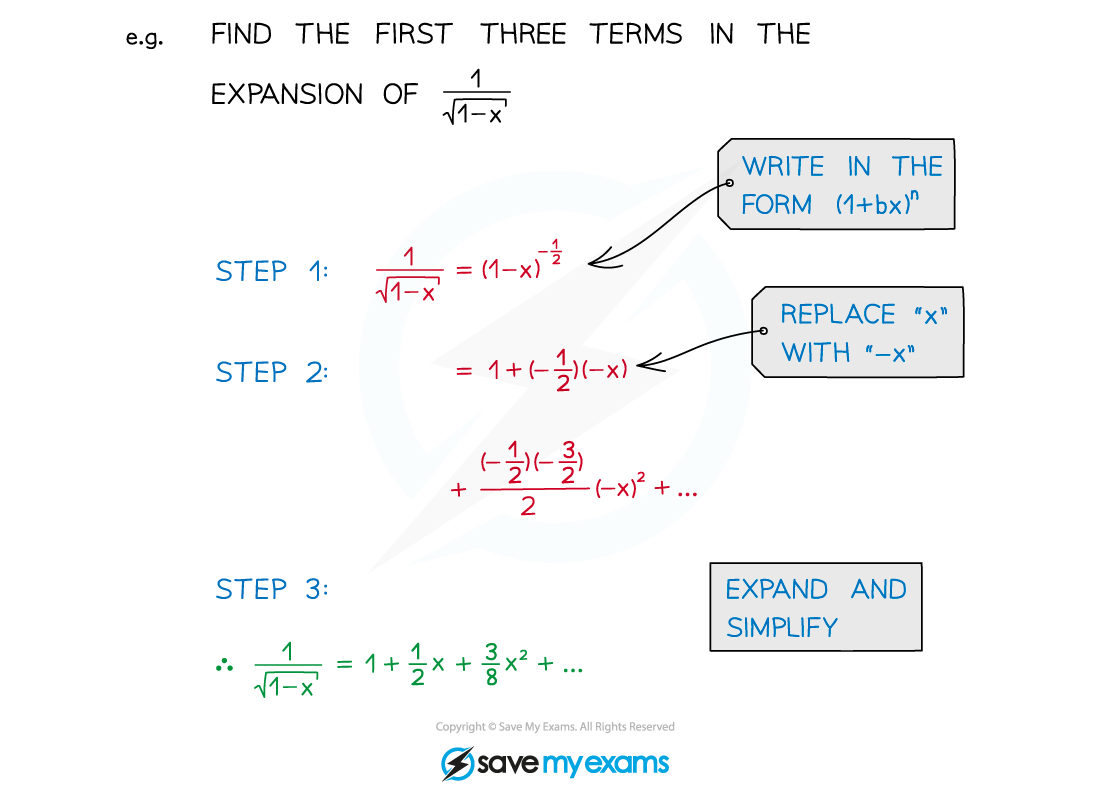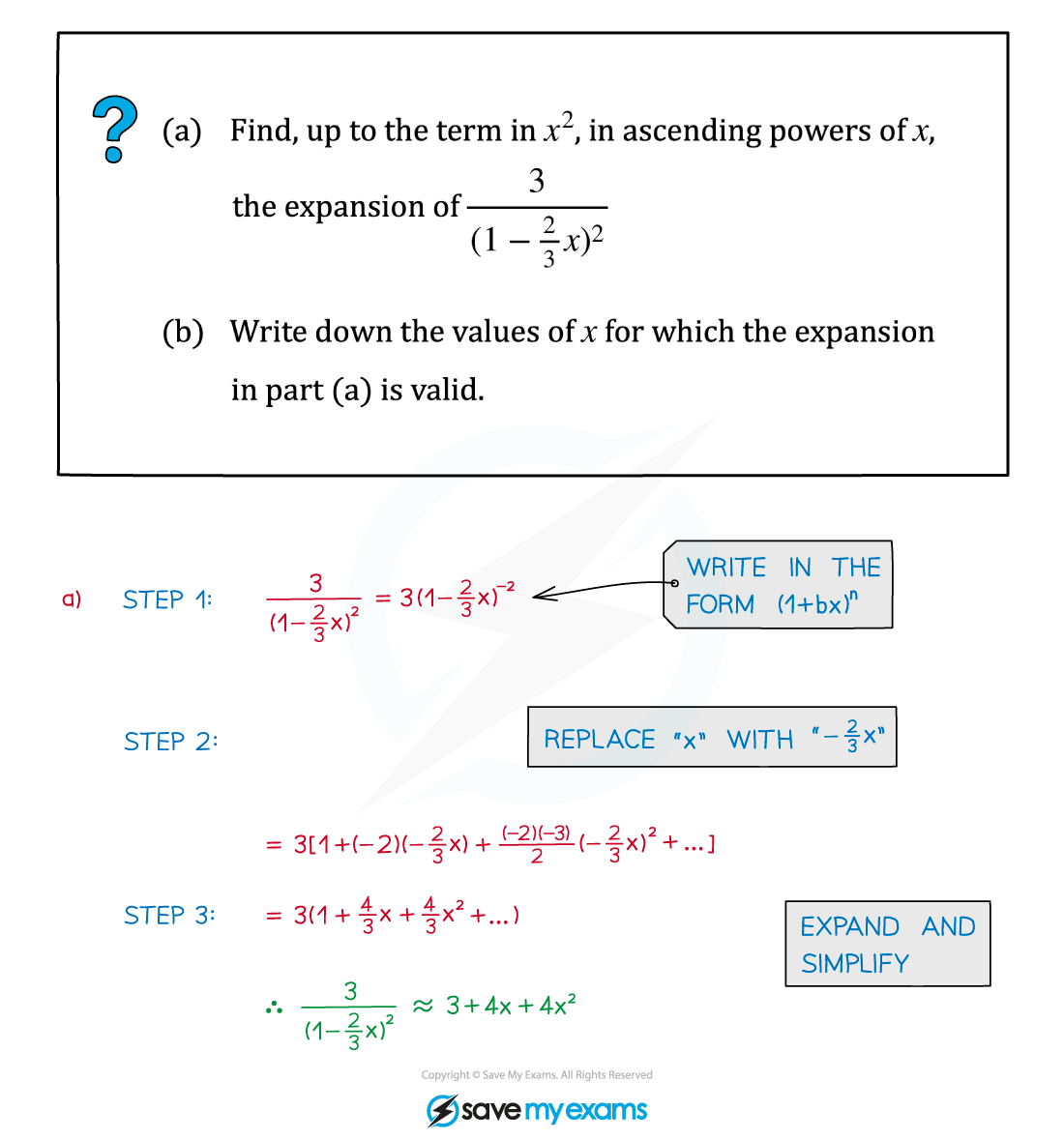# AQA A Level Maths: Pure复习笔记4.2.1 General Binomial Expansion

### General Binomial Expansion

#### What is the general binomial expansion?

• The binomial expansion applies for positive integers, n ∈ ℕ
• The general binomial expansion applies to other types of powers too• The general binomial expansion applies for all real numbers, n ∈ℝ
• Usually fractional and/or negative values of n are used
• It is derived from (a + b)n, with a = 1 and b = x
• a = 1 is the main reason the expansion can be reduced so much• Unless n ∈ ℕ, the expansion is infinitely long
• It is only valid for |x| < 1
• This is another way of writing -1 < x < 1
• This is often called the validity statement
• The restriction |x| < 1 means the series will converge
• Higher powers of x can be ignored (as r → ∞, xr → 0)
• Only the first few terms of an expansion are needed

#### How do I expand brackets with the general binomial expansion?

STEP 1       Write the expression in the form (1 + x)n
STEP 2       Expand and simplify

Use a line for each term to make things easier to read and follow

Use brackets - fractions and negatives get ugly!

STEP 3       If required, check and state the validity statement#### How do I use the general binomial expansion when it is (1 + bx)?

STEP 1       Write the expression in the form (1 + bx)n
STEP 2       Replace “x” by “bx” in the expansion

Check carefully to see if b is negative

STEP 3       Expand and simplify

Use a line for each term to make things easier to read and follow

Use brackets

STEP 4       If required, check and state the validity statement
The validity statement changes

Replace “x” with “bx” so now |bx| < 1#### Worked Example